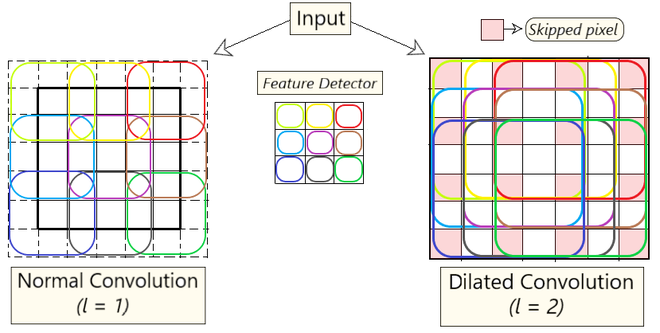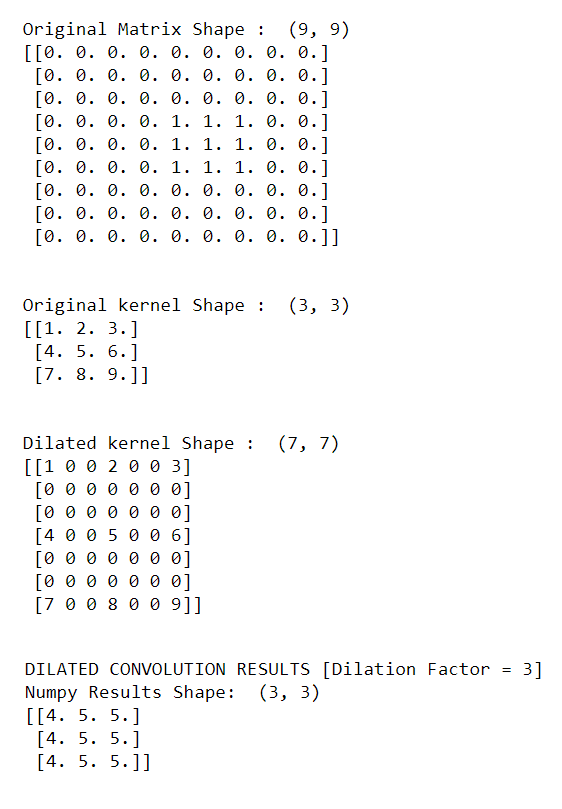Related Articles

# Dilated Convolution

• Last Updated : 30 May, 2021

Prerequisite: Convolutional Neural Networks

Dilated Convolution: It is a technique that expands the kernel (input) by inserting holes between the its consecutive elements. In simpler terms, it is same as convolution but it involves pixel skipping, so as to cover a larger area of the input.

An additional parameter l (dilation factor) tells how much the input is expanded. In other words, based on the value of this parameter, (l-1) pixels are skipped in the kernal. Fig 1 depicts the difference between normal vs dilated convolution. In essence, normal convolution is just 1-dilated convolution.Fig 1: Normal Convolution vs Dilated Convolution

Intuition:

Dilated convolution helps expand the area of the input image covered without pooling. The objective is to cover more information from the output obtained with every convolution operation. This method offers a wider field of view at the same computational cost. We determine the value of the dilation factor (l) as by seeing how much information is obtained with each convolution on varying values of l.

By using this method, we are able to obtain more information without increasing the number of kernel parameters. In Fig 1, the image on the left depicts dilated convolution. On keeping the value of l = 2, we skip 1 pixel (l – 1 pixels) while mapping the filter onto the input, thus covering more information in each step.

Formula Involved:where,
F(s) = Input
k(t) = Applied Filter
*l = l-dilated convolution
(F*lk)(p) = Output

Using this method rather than normal convolution is better as:

1. Larger receptive field (i.e. no loss of coverage)
2. Computationally efficient (as it provides a larger coverage on the same computation cost)
3. Lesser Memory consumption (as it skips the pooling step) implementation
4. No loss of resolution of the output image (as we dilate instead of performing pooling)
5. Structure of this convolution helps in maintaining the order of the data.

Code Implementation:

## Python3

 import numpy as npimport tensorflow as tfimport sysfrom  scipy.signal import convolve2d  np.random.seed(678)tf.random.set_seed(6789)sess = tf.compat.v1.Session()  # Initializing a 9x9 matrix of zeros.mat_size = 9matrix = np.zeros((mat_size,mat_size)).astype(np.float32)   # Assigning 1's in the middle of matrix # to create a random input matrixfor x in range(4,7):    for y in range(3,6):        matrix[y,x] = 1  # Creating a random kernal for testkernel = np.array([    [1,2,3],    [4,5,6],    [7,8,9]]).astype(np.float32)   print("Original Matrix Shape : ",matrix.shape)print(matrix)print('\n')print("Original kernel Shape : ",kernel.shape)print(kernel)  # self-initializing a dilated kernal.# ======[dilation factor = 3]======dilated_kernel = np.array([    [1,0,0,2,0,0,3],    [0,0,0,0,0,0,0],    [0,0,0,0,0,0,0],    [4,0,0,5,0,0,6],    [0,0,0,0,0,0,0],    [0,0,0,0,0,0,0],    [7,0,0,8,0,0,9]])  print('\n')print("Dilated kernel Shape : ",dilated_kernel.shape)print(dilated_kernel)  print('\n')print("DILATED CONVOLUTION RESULTS [Dilation Factor = 3]")output = convolve2d(matrix,dilated_kernel,mode='valid')print("Numpy Results Shape: ",output.shape)print(output)

Output

The following output is obtained from the above code.Output

The output obtained is for a dilation factor of 3. For more experimentation, you can initialize the dilated_kernel with different values of Dilation factor and observe the changes in the output obtained.

Attention reader! Don’t stop learning now. Get hold of all the important Machine Learning Concepts with the Machine Learning Foundation Course at a student-friendly price and become industry ready.

My Personal Notes arrow_drop_up